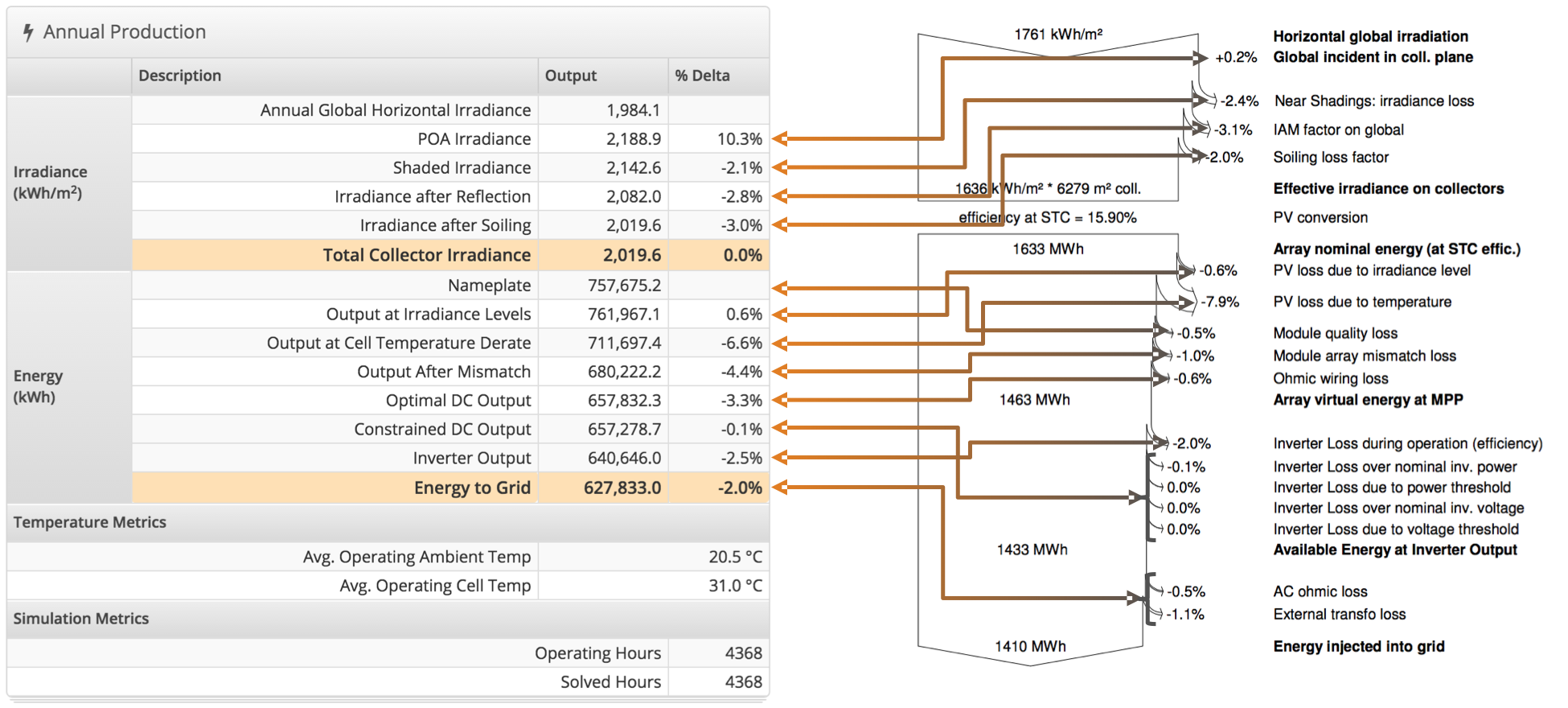# HelioScope vs. PVsyst

### Introduction

HelioScope and PVsyst use nearly all of the same equations when calculating the energy yield of a PV array. This equivalence has been verified by a third-party engineering firm, DNV GL (formerly BEW Engineering), confirming that the two models are within 1% at each step of the calculation. The primary difference between the two is that HelioScope calculates the system behavior at the module level, whereas PVsyst calculates behavior at the array level.

This document is designed to help designers align the two models, and troubleshoot situations where they do not match up.

### Aligning the Assumptions

The first step to aligning HelioScope and PVsyst is to make sure that the assumptions are similar. Most of the modeling assumptions are embedded in the Condition Sets. The key assumptions to verify are:

1. Ensure that the system design assumptions (tilt, azimuth, row spacing, near shadings) are identical
2. Ensure that the weather files are the same. Different weather sources, even for similar locations, can have differences of up to 5-10% in total available sunlight
3. Ensure that the module PAN files are identical. PAN files can be uploaded to HelioScope here: https://helioscope.folsomlabs.com/library/modules/upload
4. Make sure that the Temperature model in HelioScope is set to “Diffusion Model” – note this is different from the default, which is the Sandia model. Then click “Edit model Coefficients” to verify that the temperature coefficients are identical to PVsyst
5. Verify that the other Condition Set assumptions (transposition model, soiling assumptions, AC losses) are consistent across both models

### Aligning the Loss Tables

The loss tables of HelioScope and PVsyst can be lined up for comparison:Note that “IAM” (incident angle modifier) losses in PVsyst correspond to “Reflection” losses in HelioScope.

### Differences between the models

PVsyst and HelioScope are not identical; there are a few small differences between the models:

1. HelioScope calculates mismatch based on the individual behavior of each module and the circuit effects. PVsyst applies a loss for mismatch based on user-defined derates.
2. HelioScope calculates wiring losses based on the resistance and current of each conductor every hour. PVsyst applies a loss factor for wiring based on user-defined derates.
3. HelioScope does not specifically adjust for light-induced degradation (LID). Instead, the soiling losses can be adjusted to account for the losses or gains from LID.
4. HelioScope does not incorporate module-level adjustments to the IAM/reflection coefficients. Instead, the coefficient b~0~ has a value of 0.05 across all modules.
5. HelioScope assigns 180 degrees for South facing Azimuth, while PVsyst is 0 deg.

The models outputs are not guaranteed to always be aligned, so there are troubleshooting steps that can be taken to align the outputs: